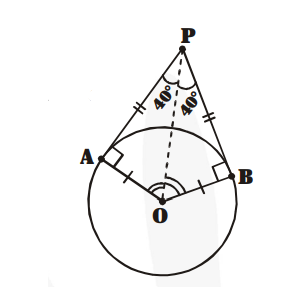# If tangents PA and PB from a point P to a circle with centre O are inclined to each other at angle of 80°,

Question:

If tangents PA and PB from a point P to a circle with centre $\mathrm{O}$ are inclined to each other at angle of $80^{\circ}$, then $\angle \mathrm{POA}$ is equal to

(A) 50°

(B) 60°

(C) 70°

(D) 80°

Solution:

In figure,

$\Delta \mathrm{OAP} \cong \Delta \mathrm{OBP}(\mathrm{SSS}$ congruence $)$

$\Rightarrow \angle \mathrm{POA}=\angle \mathrm{POB}$$=\frac{\mathbf{1}}{\mathbf{2}} \angle \mathrm{AOB}$...(1)

Also $\angle \mathrm{AOB}+\angle \mathrm{APB}=180$

$\Rightarrow \angle \mathrm{AOB}=100^{\circ} \ldots(2)$

Then from (1) and (2)

$\angle \mathrm{POA}=\frac{\mathbf{1}}{\mathbf{2}} \times .100=50^{\circ}$

Hence, the correction option is (A)